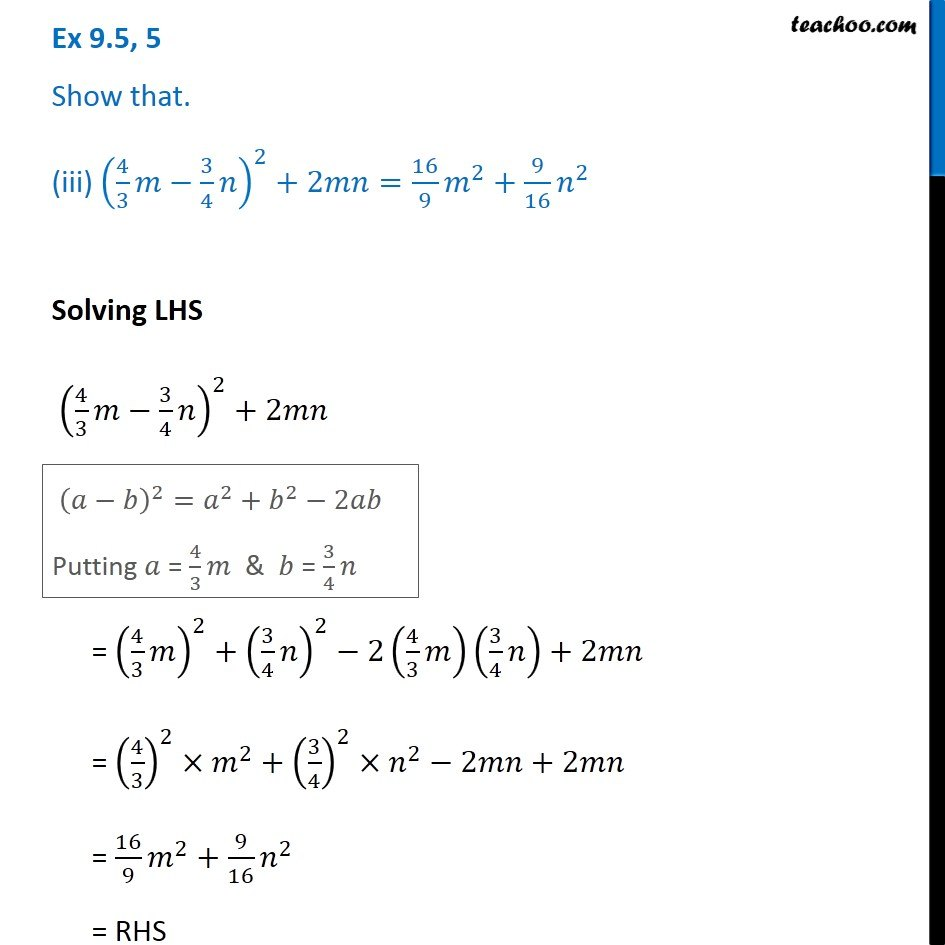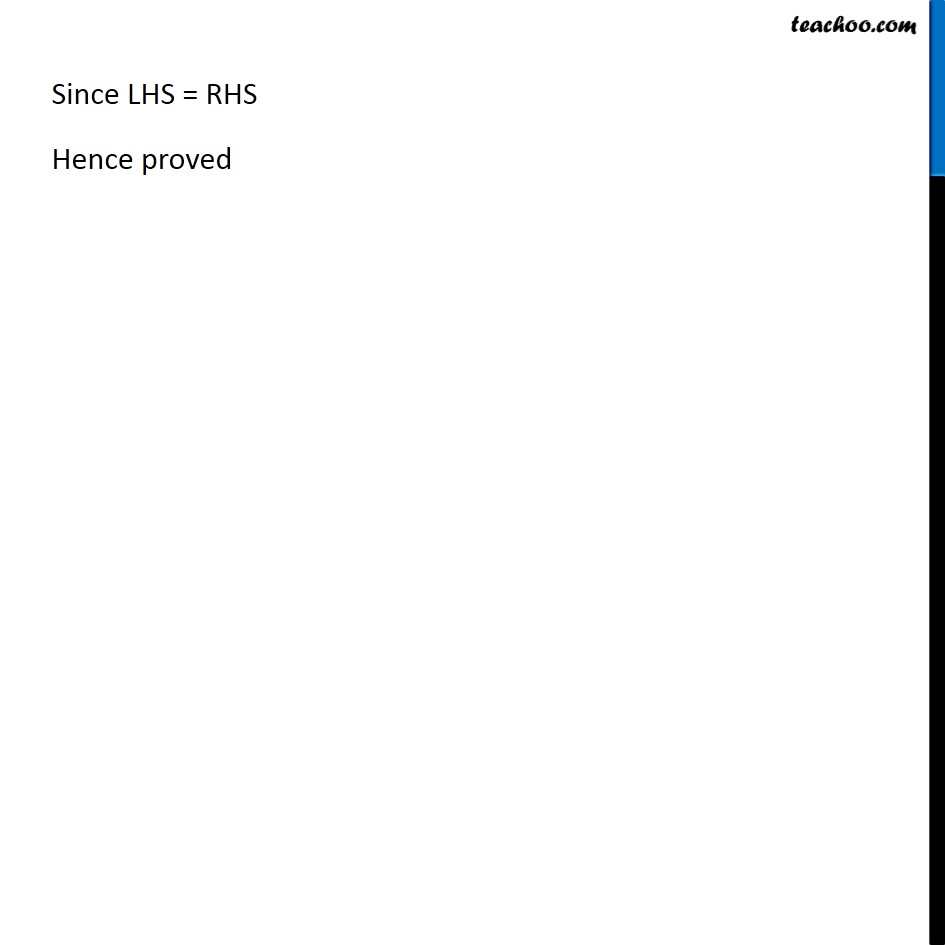Algebra Identities and Formulas

Chapter 8 Class 8 Algebraic Expressions and Identities
Serial order wiseLearn in your speed, with individual attention - Teachoo Maths 1-on-1 Class

### Transcript

Question 5 Show that. (iii) (4/3 𝑚−3/4 𝑛)^2+2𝑚𝑛=16/9 𝑚^2+9/16 𝑛^2 Solving LHS (4/3 𝑚−3/4 𝑛)^2+2𝑚𝑛 (𝑎−𝑏)^2=𝑎^2+𝑏^2−2𝑎𝑏 Putting 𝑎 = 4/3 𝑚 & 𝑏 = 3/4 𝑛 = (4/3 𝑚)^2+(3/4 𝑛)^2−2(4/3 𝑚)(3/4 𝑛)+2𝑚𝑛 = (4/3)^2×𝑚^2+(3/4)^2×𝑛^2−2𝑚𝑛+2𝑚𝑛 = 16/9 𝑚^2+9/16 𝑛^2 = RHS Since LHS = RHS Hence proved